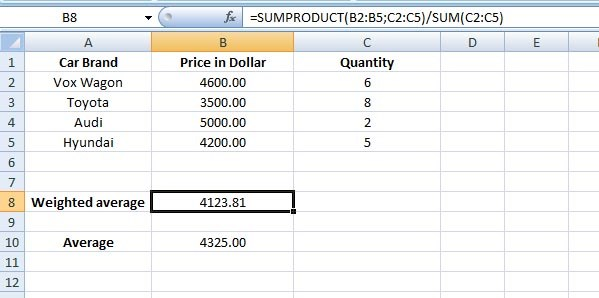## How to calculate weighted average in Excel?

Forum to talk about all the latest technologies - ins and outs!!
Admin
Site Admin
Posts: 291
Joined: Tue Sep 25, 2007 12:41 pm

### How to calculate weighted average in Excel?

An average is the sum of your data points divided by the number of data points. Here each data point contributes equally to the final average, while in weighted average, some data points contribute more than others.

Let's say, you buy and sell second-hand cars. So your recent purchase is as follows: you bought 8 Toyota cars each \$3500 and so on.

So the average price of cars = (4600 + 3500 + 5000 + 4200)/4 = 4325 dollar

But you want to get more accurate representation of the average price, so you want to calculate weighted average.In Excel you can do it by the SUMPRODUCT and SUM functions

=SUMPRODUCT(B2:B5, C2:C5) / SUM(C2:C5)

So the average price you paid per car is 4123.81 Dollar (weighted average).

How it works?

The SUMPRODUCT function is used to multiply each of the car price by its quantity (or weight), and then the total sum of it.

The SUM function is used to add up the quantities (or weights) and then the total sum of it.

Finally you divide the result of SUMPRODUCT by the SUM to get your weighted average.

In simple mathematics, you can do it as follows:

weighted average = ((4600.00 * 6) + (3500.00 * 8 ) + (5000.00 * 2) + (4200.00 * 5)) / (6 + 8 + 2 + 5) = 4123.81

So that's it.

*Recommended:

Read wonderful articles (English & German) on Science & Tech, Environment, Health and many other topics only on BlogArena.

For comments of suggestions, please contact us: info@shamskm.com